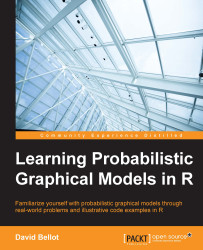•#### Learning Probabilistic Graphical Models in R#### Overview of this book

Probabilistic graphical models (PGM, also known as graphical models) are a marriage between probability theory and graph theory. Generally, PGMs use a graph-based representation. Two branches of graphical representations of distributions are commonly used, namely Bayesian networks and Markov networks. R has many packages to implement graphical models. We’ll start by showing you how to transform a classical statistical model into a modern PGM and then look at how to do exact inference in graphical models. Proceeding, we’ll introduce you to many modern R packages that will help you to perform inference on the models. We will then run a Bayesian linear regression and you’ll see the advantage of going probabilistic when you want to do prediction. Next, you’ll master using R packages and implementing its techniques. Finally, you’ll be presented with machine learning applications that have a direct impact in many fields. Here, we’ll cover clustering and the discovery of hidden information in big data, as well as two important methods, PCA and ICA, to reduce the size of big problems.
Learning Probabilistic Graphical Models in RCreditswww.PacktPub.comPrefaceFree Chapter
Probabilistic ReasoningExact InferenceLearning ParametersBayesian Modeling – Basic ModelsApproximate InferenceBayesian Modeling – Linear ModelsProbabilistic Mixture ModelsAppendixIndex## Sampling from a distribution

We have a big problem with probabilistic graphical models in general: they are intractable. They quickly become so complex that it is impossible to run anything in a reasonable amount of time. Not to mention learning them. Remember, for a simple algorithm such as EM, we need to compute a posterior distribution at each iteration. If the dataset is big, which is common now, if the model has a lot of dimensions, which is also common, it becomes totally prohibitive. Moreover, we limited ourselves to a small class of distributions, such as multinomial or Gaussian distributions. Even if they can cover a wide range of applications, it's not the case all the time.

In this chapter, we consider a new class of algorithms based on the idea of sampling from a distribution. Sampling here means to draw values of the parameters at random, following a particular distribution. For example, if one throws a dice, one draws a sample from a multinomial distribution, such that six values...